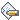# Equilibrium 2

2 NOCl (g)<- ->2 NO (g)+Cl2 (g) Kp = 6.8x10^-19 at 590.0K Delta H^o = +276.3kJ/mol
a) Write the equilibrium expression for the pressure constant, Kp.
b) Gaseous NOCl is added to an evacuated 1.23 L container kept at 590.0K until the pressure inside the container reaches 1.15 atm. Find the partial pressures of each gas when the system reaches equilibrium.
c) Find Kc for this process at 590.0K.
d) Find the change in free energy, Delta G^o 590.0K, for this system at 590.0 K.
e) Does this system contain mostly reactants or mostly products at equilibrium? Justify your answer.
f) In what way can the system be manipulated in order to increase the value of the equilibrium constant, Kp? Justify your answer.
g) What is the equilibrium constant, Kp, for 2 NO (g) + Cl2 (g) <- -> 2 NOCL (g) at 590.0 K? Justify your answer with calculations.
h) Suppose the process on the system was increased. In which direction would the reaction proceed? Justify your answer.
i) The three gases were injected into a separate container at 590.0K and their partial pressures were found to be P (NOCl) =1.2 atm, P(Cl2) = 1.2 atm, and P(NO) = 0.19 atm. Is the system at equilibrium? If not, in which direction is the reaction proceeding? Justify your answers.
1) Kp you need to determine from balanced reaction
kp = (p No)^2*pCl2  /p(NOCl)^2
2.
Initial pressure of NOCl is given
we can make ICE table
2NOCl  <--->  2NO + Cl2
I       1.15                  0         0
C      -2x                    +2x     + x
E       1.15-2x             2x         x
Now plug in these E partial pressure vlaues in the kp expression  and solve it for kp
C
Kp = Kc (RT)^(delta n)
delta n =  n(moles of products)-n(moles of reactants)
= 3-2 =1
Get the value of kc
R is the universal gas constant and T is the temperature in kelvin
d).delta G = -RT ln Kc
e) kp value is less than 1 and very small.This indicates products are very less.
plug in the values of R in kJ/mol.K and Kc from c part
f).Kp is the equilibrium constant and only temperature can change its value .Reaction is endothermic and if you increase temperature it will shift it more to the  product side.
g).If we reverse the equation kp value will get inversed.
new kp = 1/( 6.8x10^-19)
h) which process???
i)Calculate Qc and compare it with Kc
if Qc> Kc then equilibrium will shift to left and if Qc<Kc then it will shift to the right.